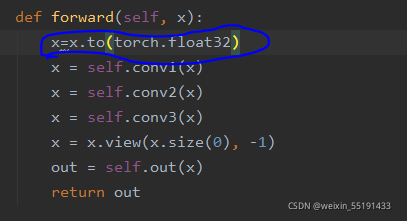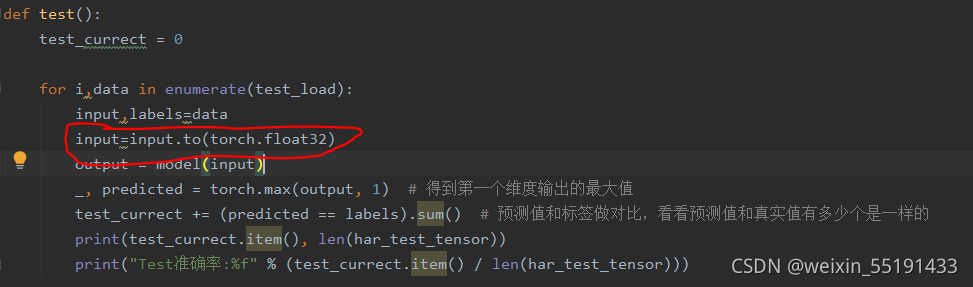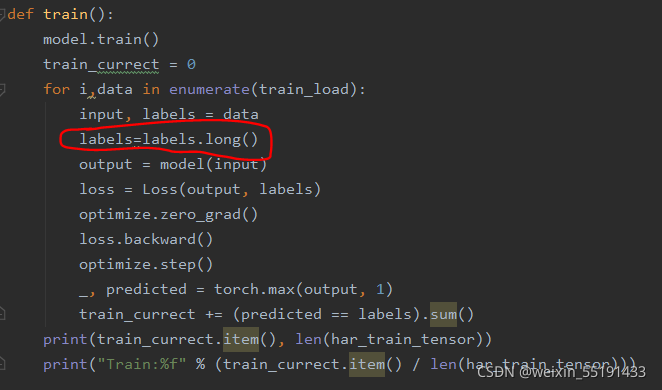# Pytorch Error: runtimeerror: expected scalar type double but found float

The reason for this problem may be that the data type of tensor is wrong, it may be the wrong type of input X in the backpropagation, or the wrong data type in the training and testing process. If it is the backpropagation process, it depends on which layer of neural network has the problem, and add x = x.to (torch. Float32) in front of which layer, For example, this problem occurs in the first layer of neural network. For example, this problem occurs in the first layer of convolution. The solution is as follows:If this problem occurs in the training or test model, the solution is as follows:It is also possible that the type of labels is wrong during training or testing. The solutions are as follows: